Physiolibrary.Blocks.Math

Modelica.Math extension

Information

Extends from Modelica.Icons.Package (Icon for standard packages).

Package Content

Name Description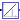Integrator Integrator with support of steady state calculation.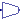Add u + parameter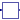Reciprocal 1 / u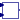Power b ^ uMin Pass through the smallest signal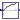Log10AsEffect min( 0, log10(u) )Parts Divide the input value by weightsHomotopyStrongComponentBreaker Break the strong component in normalized signal with independent default constant value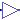DegradationGain Output the degradation flow from HalfTime and the amount as the input signalFractionGain Output the fraction of the input signal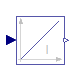Physiolibrary.Blocks.Math.Integrator

Integrator with support of steady state calculation.Information

This blocks computes output y (element-wise) as integral of the input u multiplied with the gain k:

k
y = - u
s

It might be difficult to initialize the integrator in steady state. This is discussed in the description of package Continuous.

Extends from SteadyStates.Interfaces.SteadyState (Abstract class for any dynamic state calculation (for any derivation), which is driven by SimulationType option.), Modelica.Blocks.Interfaces.SISO (Single Input Single Output continuous control block).

Parameters

TypeNameDefaultDescription
Realk1Integrator gain
Initialization
Realy_start0Initial or guess value of output (= state)
IO
replaceable package UtilitiesTypes.FilesUtilities (direct...How to store or load the values
Initialization
Realstate_starty_startState start or init value
Value I/O
StringstoreUnit""Unit in Utilities input/output function
StringstateNamegetInstanceName()Name in Utilities input/output function
BooleanSAVE_RESULTSfalseSave final state values
BooleanSAVE_COMPARISONfalseCompare final state values with original values
Equilibrium
Simulation
SimulationTypeSimulationTypes.SimulationType.NormalI...Dynamic with Initialization or Steady State
BooleanisDependentfalse=true, If zero flow is propagated in eqiulibrium through resistors, impedances, reactions, etc.
Solver
Numerical support of very small concentrations
RealNominalValue1Numerical scale. For some substances such as hormones, hydronium or hydroxide ions should be set.

Modelica definition

model Integrator "Integrator with support of steady state calculation." extends SteadyStates.Interfaces.SteadyState( state_start=y_start, state(nominal=NominalValue)); parameter Real k=1 "Integrator gain"; parameter Real y_start=0 "Initial or guess value of output (= state)"; extends Modelica.Blocks.Interfaces.SISO(u(nominal=NominalValue/k),y(nominal=NominalValue)); parameter Real NominalValue = 1 "Numerical scale. For some substances such as hormones, hydronium or hydroxide ions should be set."; equation state = y; //der(y) = k*u change = k*u; end Integrator;Physiolibrary.Blocks.Math.Add

u + parameterInformation

This block computes output y as sum of offset k with the input u:

y = k + u;

Parameters

TypeNameDefaultDescription
Realk value added to input signal

Connectors

TypeNameDescription
input RealInputuInput signal connector
output RealOutputyOutput signal connector

Modelica definition

block Add "u + parameter" parameter Real k(start=1) "value added to input signal"; public Modelica.Blocks.Interfaces.RealInput u "Input signal connector"; Modelica.Blocks.Interfaces.RealOutput y "Output signal connector"; equation y = k+u; end Add;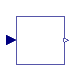Physiolibrary.Blocks.Math.Reciprocal

1 / uInformation

This blocks computes the output y as reciprocal value of the input u:

y = 1 / u ;

Extends from Modelica.Blocks.Interfaces.SISO (Single Input Single Output continuous control block).

Connectors

TypeNameDescription
input RealInputuConnector of Real input signal
output RealOutputyConnector of Real output signal

Modelica definition

block Reciprocal "1 / u" extends Modelica.Blocks.Interfaces.SISO; equation y = 1/u; end Reciprocal;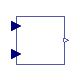Physiolibrary.Blocks.Math.Power

b ^ uInformation

y = base^exponent

Parameters

TypeNameDefaultDescription
RealBase10exponential base if useBaseInput=false
External inputs/outputs
BooleanuseBaseInputfalse=true, if exponential base input is used instead of parameter Base

Connectors

TypeNameDescription
output RealOutputy
input RealInputbase
input RealInputexponent

Modelica definition

block Power "b ^ u" parameter Boolean useBaseInput = false "=true, if exponential base input is used instead of parameter Base"; parameter Real Base=10 "exponential base if useBaseInput=false"; Modelica.Blocks.Interfaces.RealOutput y; Modelica.Blocks.Interfaces.RealInput base(start=Base) = b if useBaseInput; Modelica.Blocks.Interfaces.RealInput exponent; protected Real b "Current exponential base"; equation if not useBaseInput then b = Base; end if; y = b^exponent; end Power;Physiolibrary.Blocks.Math.Min

Pass through the smallest signalInformation

This block computes the output y as minimum of the Real inputs u,u .. u[nin]:

y = min ( u );

Extends from Modelica.Blocks.Interfaces.MISO (Multiple Input Single Output continuous control block).

Parameters

TypeNameDefaultDescription
Integernin1Number of inputs

Connectors

TypeNameDescription
input RealInputu[nin]Connector of Real input signals
output RealOutputyConnector of Real output signal

Modelica definition

block Min "Pass through the smallest signal" extends Modelica.Blocks.Interfaces.MISO; equation y = min(u); end Min;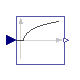Physiolibrary.Blocks.Math.Log10AsEffect

min( 0, log10(u) )Information

This blocks computes the output y as the base 10 logarithm of the input u if u>1 or 0 otherwise

y = if(u>1) log10( u ) else 0;

Extends from Modelica.Blocks.Interfaces.SISO (Single Input Single Output continuous control block).

Connectors

TypeNameDescription
input RealInputuConnector of Real input signal
output RealOutputyConnector of Real output signal

Modelica definition

block Log10AsEffect "min( 0, log10(u) )" extends Modelica.Blocks.Interfaces.SISO; equation y = if u>1 then Modelica.Math.log10(u) else 0; end Log10AsEffect;Physiolibrary.Blocks.Math.Parts

Divide the input value by weightsInformation

This blocks divide input value u to output array y by weights. The sum of output values is equal to input value u:

u = (w*y + w*y + ... + w[n]*y[n]) / (w + w + ... + w[n]);

Example:

parameter:   nin = 3;  w=ones(3);

results in the following equations:

y=u/3, y=u/3, y=u/3;

Extends from Modelica.Blocks.Interfaces.SIMO (Single Input Multiple Output continuous control block).

Parameters

TypeNameDefaultDescription
Integernout1Number of outputs
Realw[nout]ones(nout)Optional: weight coefficients

Connectors

TypeNameDescription
input RealInputuConnector of Real input signal
output RealOutputy[nout]Connector of Real output signals

Modelica definition

block Parts "Divide the input value by weights" extends Modelica.Blocks.Interfaces.SIMO; parameter Real w[nout]=ones(nout) "Optional: weight coefficients"; protected Real coef; Real weight[nout]; equation ones(nout)*weight = 1; for i in 1:nout loop weight[i] = w[i] * coef; y[i] = u * weight[i]; end for; end Parts;Physiolibrary.Blocks.Math.HomotopyStrongComponentBreaker

Break the strong component in normalized signal with independent default constant valueInformation

This blocks should solve the initial strong component problem. In the non-linear-strong-component-cycled place, where the default or mean value of variable is known.

For example the regulation loop L driven by loop-dependent effect E with default value 1:

E=f(L(E)) can be rewritten to E=f(L( H )), where H is output from this block with input E.

Extends from Modelica.Blocks.Interfaces.SISO (Single Input Single Output continuous control block).

Parameters

TypeNameDefaultDescription
RealdefaultValue1
RealdefaultSlope0

Connectors

TypeNameDescription
input RealInputuConnector of Real input signal
output RealOutputyConnector of Real output signal

Modelica definition

block HomotopyStrongComponentBreaker "Break the strong component in normalized signal with independent default constant value" extends Modelica.Blocks.Interfaces.SISO; parameter Real defaultValue=1; parameter Real defaultSlope=0; equation y = homotopy(u,defaultValue + defaultSlope*(u-defaultValue)); //y = homotopy(u,defaultValue); end HomotopyStrongComponentBreaker;Physiolibrary.Blocks.Math.DegradationGain

Output the degradation flow from HalfTime and the amount as the input signalInformation

This block computes output y as product of gain k with the input u:

y = k * u;

Parameters

TypeNameDefaultDescription
TimeHalfTime Half time to compute degradation from amount or mass [s]

Connectors

TypeNameDescription
input RealInputuInput signal connector
output RealOutputyOutput signal connector

Modelica definition

block DegradationGain "Output the degradation flow from HalfTime and the amount as the input signal" parameter Types.Time HalfTime "Half time to compute degradation from amount or mass"; public Modelica.Blocks.Interfaces.RealInput u "Input signal connector"; Modelica.Blocks.Interfaces.RealOutput y "Output signal connector"; equation y = (Modelica.Math.log(2)/HalfTime)*u; end DegradationGain;Physiolibrary.Blocks.Math.FractionGain

Output the fraction of the input signalInformation

This block computes output y as product of gain k with the input u:

y = k * u;

Parameters

TypeNameDefaultDescription
Fractionf Half time to compute degradation from amount or mass 

Connectors

TypeNameDescription
input RealInputuInput signal connector
output RealOutputyOutput signal connector

Modelica definition

block FractionGain "Output the fraction of the input signal" parameter Types.Fraction f "Half time to compute degradation from amount or mass"; public Modelica.Blocks.Interfaces.RealInput u "Input signal connector"; Modelica.Blocks.Interfaces.RealOutput y "Output signal connector"; equation y = f*u; end FractionGain;

Automatically generated Tue Sep 15 22:54:48 2015.